American Journal of Theoretical and Applied Statistics
Volume 5, Issue 4, July 2016, Pages: 162-172

Determinants of Environmental Health Related Diseases in Kenya with Generalized Linear Mixed Models: Analysis of Kenya Integrated Household Budget Survey

Jemimah Wangui Muraya1, Beatrice Karanja Kimani1, John Mwangi Ndiritu2

1Department of Statistics and Computer Science, Moi University, Eldoret, Kenya

2School of Mathematics, University of Nairobi, Nairobi, Kenya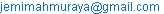(J. W. Muraya)(Beatrice K. K.)(J. M. Ndiritu)

Jemimah Wangui Muraya, Beatrice Karanja Kimani, John Mwangi Ndiritu. Determinants of Environmental Health Related Diseases in Kenya with Generalized Linear Mixed Models: Analysis of Kenya Integrated Household Budget Survey. American Journal of Theoretical and Applied Statistics. Vol. 5, No. 4, 2016, pp. 162-172. doi: 10.11648/j.ajtas.20160504.11

Received: February 29, 2016; Accepted: March 11, 2016; Published: June 4, 2016

Abstract: Generalized linear models (GLMs) form a class of fixed effects regression models for several types of dependent variable, whether continuous, dichotomous or counts. Common GLMs include linear regression, Logistic regression and Poison regression. These models have typically been used a lot in modeling of data arising from a heterogeneous population under the assumption of independence. However, in applied science and in real life situations in general, one is confronted with collection of correlated data (Mark Aerts et al, 2005). This generic term embraces a multitude of data structures, such as multivariate observations, clustered data, repeated measurements, longitudinal data, and spatially correlated data. Generalized Linear Mixed Models (GLMMs) are able to handle extraordinary range of complications in regression-type analyses. They are often used to handle correlations that arise in longitudinal and other clustered data. This study sought to fit GLMMs to Kenya integrated household data collected in 2005/6 to explain different factors and their influence on an individual morbidity in Kenya. The cluster variable was used to introduce the random effect in this data. From the analysis, it was deduced that gender increases the log-odds of an individual getting a disease, while people who are living in good housing conditions reduces the log-odds of an individual experiencing morbidity. Main source of drinking water and the human waste disposal method were significant in explaining individual morbidity in Kenya. This study can however be extended to incorporate other factors such as income level of individuals. Individuals with low level of income are believed to be more likely to experience environmental health related diseases than individuals with higher levels of income.

Keywords: Generalized Linear Mixed Effects Model GLMEM, Maximum Likelihood ML, Restricted Maximum Likelihood REML, Marginal Quasi Likelihood MQL, Demographic and Health Surveys DHS, Deviance Information Criteria DIC, Akaike Information Criteria AIC

1. Introduction

1.1. Background of the Study

Generalized linear mixed models (GLMMs) continue to grow in popularity due to their ability to directly acknowledge multiple levels of dependency and model different data type. GLMMs extend the generalized linear model, as proposed by Nelder and Wedderburn (1972) and comprehensively described in Mc Cullaghand Nelder (1989), by adding normally distributed random effects on the linear predictor scale in order to include the concept of correlated data such as clustered data.

GLMM is one of the most useful structures in modern statistics, allowing many complications to be handled within the familiar linear model framework. The fitting of such models has been the subject of a great deal of research over the past decade. Early contributions to fitting various forms of the GLMM include Stiratelli, Laird and Ware (1984), Anderson and Aitkin (1985), Gilmour, Anderson and Rae (1985), Schall (1991), and Breslow and Clayton (1993).

Most literature on GLMM is around grouped data. For any model, parameter estimation is always one of the most important aspects of statistical inference. Many researchers have made efforts to estimate parameters using GLMMs. For instance, Hall; Hall, (2000) applied Maximum Likelihood (ML) estimation and Yau and Lee, (2001) applied hierarchical likelihood method of estimation to zero-inflated (ZI) mixed models. In this project, ML for normal random effect of GLMMs and Restricted maximum likelihood (REML) method when assuming random effect distribution is unknown will be used.

This study seeks to fit generalized linear mixed effects model to house hold data that was collected in 2005/6. In this survey, clusters were randomly selected across all the districts in Kenya. In each selected cluster, households were randomly selected with equal probability in each cluster; members in the selected households were interviewed. This study therefore proposes that cluster variable will introduce the random effect in this data. It is assumed that members in the same cluster are more likely to experience similar morbidity structures compared to members in different clusters.

1.2. Literature Review

Generalized linear mixed effects models have been used for long time and more so by epidemiologists in the analysis of dichotomous data. Most of the recent contributions to the use of GLMMs was a study by Kandala, Nyovani, (2004). Their study aimed at describing the spatial variation in the prevalence of diarrhea, cough and fever among children under 5 years using the 1992 Demographic and Health surveys (DHS) of Malawi and Zambia. Individual data record was constructed for 3660 children in Malawi and 5268 children in Zambia. Each record represents a child and consists of morbidity information and a list of covariates.

Geo-additive logistic analyzes was used on the probability of a child being ill with malaria, cough, and diarrhea during the preference period to determine the socio-economic, demographic variables that are associated with these three ailments while simultaneously controlling for spatial dependence in the data and possibly nonlinear effects of covariates.

The response variable applied was defined as

yit=1: if a child i was ill during the preference period t

0: if a child i survive the illness,

Two models were fit in this data: simpler parametric probit model and probit model with dynamic and spatial effects for the probability of falling ill at month t.

M1: nit=X’itB

M2: nit=f1(age)+f2(mab)+funstr(dist)+fstr(dist)+X’itB

The fixed effects in model M1 included all the covariates with constant fixed effects. When the two models were compared, it turned out that model M2 was superior in terms of Deviance Information Criteria (DIC) [Spiegelhalter et. al., 2002] which is a method used for model comparison. In addition, model M2 in the DIC, accounted for the unobserved heterogeneity that might exist in the data, which cannot be captured by the covariates.

The effects of f1 and f2 were modeled by cubic penalized splines with second order random walk penalty. Spatial affects fstr(s) were experimented with different prior assumptions.

In both countries models were estimated where either a structured or an unstructured effect was included as well as a model where both effects were included. As a result there was clear evidence for both countries of spatial correlation among neighboring districts. Hence, a spatially correlated effect fstr was included into the predictors of the final models. Additionally, an unstructured effect funstr was included because there was evidence of local extra variation in the highly urbanized areas in Malawi and Zambia.

Including the spatial component funstr+fstr(dist) increases model complexity. With such model, it is assumed that random components at the contextual level (district) are mutually independent. The estimates of the presumed spatial correlated districts level random effects showed strong evidence of spatial dependence.

Hedeker and Gibbons (2003) described a random effects ordinal probit regression model, examining longitudinal data collected in the NIMHS chizophrenia Collaborative Study on treatment related changes in overall severity. The dependent variable was item 79 of the Inpatient Multidimensional Psychiatric Scale (IMPS; ), scored as: (a) normal or borderline mentally ill, (b) mildly or moderately ill, (c) markedly ill, and (d) severely or among the most extremely ill. In this study, patients were randomly assigned to receive one of four medications: placebo, chlorpromazine, ﬂuphenazine, orthioridazine.

Here, a logistic GLMM with random intercept and trend was ﬁt to these data using SAS PROC NLMIXED with adaptive quadrature. Fixed effects included a dummy-coded drug effect placebo=0 and drug=1), a time effect (square root of week; this was used to linearize the relationship between the cumulative logits and week) and a drug by time interaction.

The results indicated that the treatment groups do not signiﬁcantly differ at baseline (drug effect), the placebo group does improve overtime (signiﬁcant negative time effect), and the drug group has greater improvement overtime relative to the placebo group (signiﬁcant negative drug by time interaction). Thus, the analysis supports use of the drug, relative to placebo, in the treatment of schizophrenia. Comparing this model to a simpler random intercepts model yields clear evidence of signiﬁcant variation in both the individual intercept and time-trends likelihood-ratio.

Also, a moderate negative association between the intercept and linear time terms is indicated, expressed as a correlation it equals -.40, suggesting that those patients with the highest initial severity show the greatest improvement across time (e.g., largest negative time trends). This latter ﬁnding could be a result of a ﬂoor effect’, in that patient with low initial severity scores cannot exhibit large negative time-trends due to the limited range in the ordinal outcome variable.

There were more work on morbidity and factors associated to morbidity that involved GLMM that was done in (2002) by Narayan, Sarah B. et al, (2002). This analysis sought to examine trends and differentials in diarrhea prevalence and treatment in Brazil between 1986 and 1996 using data from Demographic and Health Survey program. Information on child health, health-related behavior, use of health care services and several other topics was collected. The survey was based on a multistage clustered sampling scheme. A total of 8,369 dwellings units was selected for the survey across 337 primary sampling units (PSUs) whereby PSUs represented the entire country. Interviews were completed with 5,892 women aged 15 to 44 years and information on diarrhea was obtained for 3,183 children born to these women.

Multilevel logistic regression was used to model the relationship between the diarrhea prevalence and the background and intermediate factors. The dependent variable was a binary response, yijk, that indicated whether the ithchild of the jth family living in the kth community had diarrhea (yijk= 1) or not (yijk =0). The probability of a child having diarrhea was defined as pijk = pr (yijk=1) and logit transformation of pijk modeled as a linear function of the covariates in the model:

Log [pijk / (1-pijk)] = X’ijkβ1+X’jkβ2+X’kβ3+ujk+vk

ujk represents a family-level random effect and vk a community-level random effect that are each normally distributed with a zero mean and variance δu2 and δv2 respectively. Xijk represents background child covariates, Xjk family covariates and Xk community covariates.

Model below included intermediate child covariates (Wijk) and intermediate family covariates (Wjk).

Log [pijk / (1-Pijk)] = W’ijkγ1 + W’jkγ2 + X’ijkβ1+ X’jkβ2 + X’k β3 + ujk + vk

The two models above allowed them study how background factors directly and indirectly affected diarrhea prevalence. The first model showed the total effect of each background factor on diarrhea prevalence.

This study showed that the family and the community random effects were statistically significant in both models; although unobserved family effects were far more important than unobserved community effects. The variance of the family random effect (2.33) was more than six times as large as the variance for the cluster random effect (0.35). The intra-family level correlation was .45 while the intra-cluster correlation was only .06.

The large family-level variance indicates that there was a strong correlation in the chances of siblings having diarrhea that may be the result of important unmeasured maternal characteristics and household environmental factors (Sastry, 1997).

The study also found that here were significant effects on diarrhea of child age, mother’s education, father’s education, parent’s marital status, rural-urban place of residence, and region of residence.

More work to the use of GLMMs was a study by Gruder, Gruderet AL, (1993). This study aimed at describing smoking cessation, whereby 489 individuals were randomized into three groups; Control, discussion, or social support conditions. The control group was given a self help manual and encouraged to watch twenty television programs on smoking cessation. Subjects on the experimental groups were in addition given a chance to participate in group meetings and were given further training in support and relapse prevention. To analyze the data as binary response variables, the two experimental groups were combined together into one category called experimental group. Data were collected at four telephone interviews: post intervention, and 6, 12, and 24 months later. Smoking abstinence rates at these four times were as follows:

-Control group: =109, 97, 92,

-Experimental group: =380, 357, 337, and 295

Two logistic GLMM were fit to this data i.e. a random intercept model and a random intercept and linear trend of time model. In this study, the analysis was based on the probability of smoking abstinence and not the probability of smoking. The fixed effect were the group, with 0=control and 1=experimental. Based on a likelihood-ratio test, the random intercept and linear trend of time model was preferred (with a–2log likelihood ratio=1594.7) to the random intercept model (with a-2 log likelihood ratio=1631.0). As a result, there was a clear evidence of subjects varying by both the intercepts and the time trends. Both models had a non singular time effect, but the treatment was highly significant. Interaction between condition and time was non-significant in the both models, which suggested declining condition over time. The interaction was non-significant in the random intercepts and time trend model, but was significant in the random intercept model.

This study showed that the significance of model terms can highly depend on the structure of the random effects. Therefore, a researcher must decide upon a reasonable model for the random effects as well as for fixed effects. A recommended approach is to perform a sequential model selection procedure such as stepwise regression analysis. Here one includes all the possible covariates of interest into the model and selects between the possible models of random effects using model fit criteria such as the likelihood ratio test, Deviance analysis, Akaike Information Criteria among others. In this study, I shall take advantage of the superiority of Akaike Information Criteria of being adjusted for both the sample size and the number of parameters in the model. For model selection criterion, I shall use the backward stepwise selection, whereby the model with a smaller AIC value being preferred to the model with larger value.

Carla J. Machado and Ken Hill July (2003)  used data for the (1998)–birth cohort, City of S. Paulo, Brazil. The hypothesis was that early infant morbidity may produce adverse outcomes in subsequent life. The duo used Apgar units to estimate early infant morbidities, with a low Apgar score being a convenient measure of early infant morbidity. The study used determinants of early infant morbidity (sex, plurality, mode of delivery, prior losses, gestation age, prenatal care and birth weight, parity and maternal age, race, maternal education and community development).

Information was extracted from 2009,628 birth records, and used multivariate logistic regression to assess the effect of each independent variable on Apgar score less than seven at one minute and Apgar score less than seven at five minutes.

The outcome variable was whether or not an infant had an Apgar score below seven at one minute or not and whether or not an infant had an Apgar score below seven at five minutes. The explanatory variables were classified as;

1. Proximate determinants-birth weight, gestation age, prenatal care, sex, plurality, prior losses and mode of delivery

2. Less proximate determinates- parity and maternal age

3. Distal determinants- race, maternal education and community development

To obtain an adjusted odds ratio, a multivariate logistic regression model was used in order to model the two dichotomous outcomes. Because characteristics of mothers and infants from the same community were related, the standard errors were corrected for lack of independence between observations using the Huber/White Sandwich correction, which assumes that observations are independent across clusters but not within clusters (the community of mother’s residence at the time of birth).

From their results, Low birth weight, prematurity and community development had strong prediction of morbidity. Maternal education showed strong negative correlation with both Apgar scores. The negative correlations between maternal schooling and Apgar scores were observed after prenatal care, parity and maternal age were included in the model. Children of very young adolescent mothers had lower Apgar scores at one minute (but not at five minutes) than those born to mothers aged 15 to 19. Parity one or higher was associated with decreased odds of low Apgar scores. Cesarean section and operative delivery were also strongly associated with higher odds of early infant morbidity.

2. Methodology

2.1. Data and Variables

The data for this study comes from the Kenya Integrated Household Budget Survey (KIHBS) conducted by Kenya National Bureau of Statistics in (2005/6). In KIHBS, data was collected over a period of 12 months, which covered all possible seasons. This survey was to collect a wide spectrum of socio-economic indicators required to measure, monitor and analyze the progress made in improving living standards. The Household Questionnaire was designed to collect information on the following: demographics, housing, education, health, agriculture and livestock, enterprises, expenditure and consumption, among others.

The Survey was conducted in 1,343 randomly selected clusters across all districts in Kenya and comprised 861 rural and 482 urban clusters, 10 households were randomly selected with equal probability in each cluster resulting in a total sample size of 13,430 households. This study is confined to members of the household who experienced any sort of disease at the time of the study. This produces a data set comprising about 66,725 individuals.

Dependent Variable: The outcome variable of interest (morbidity) asked whether a member of household had suffered from environmental health related disease. This variable is binary in nature with values (1=household member had environmental health related disease, 0= household member had not experienced environmental health related disease).

Explanatory Variables: This study used explanatory variables available in the Kenya Integrated Household Budget Survey data. These include socio economic and demographic variables. The socio economic variables used in the study include gender, highest level of education, individual working status, main source of drinking water, housing condition and means of human waste disposal. The demographic variable used is area of residence i.e. rural/urban.

2.2. Exponential Distribution Family

The distribution of a random variable yi (with mean µi) is said to belong to the exponential family if it has a probability density function of the form;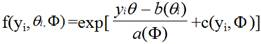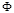is a constant dispersion parameter, θi is the natural or canonical parameter that can be expressed as some function of mean µi and kθi is a cumulant generating function. Among many of the common distributions that are known to belong to this distribution include; Normal, Gamma, Poisson and Binomial.

2.3. Generalized Linear Models (GLM’s)

The generalized linear model (GLM) refers to a larger class of models popularized by Mc Cullaghand Nelder (1982, 2nd edition 1989). In these models, the response variable yi is assumed to follow an exponential family distribution with mean μi, which is assumed to be some (often nonlinear) function of xTiβ.

They represent a class of fixed effects regression models for several types of dependent variables (i.e. continuous, dichotomous, counts). Thus, it can be said that the generalized linear model involves logistic models for binary dependent variables, log linear analysis, Poisson regression, etc.

There are three components to any GLMs:

1. Random Component– refers to the probability distribution of the response variable (Y); e.g. normal distribution for Y in the linear regression, or binomial distribution for Y in the binary logistic regression. Yi’s are independent and random variables with mean E(Yi)=µi, and are member of the exponential family of distributions.

2. Systematic Component-specifies the explanatory variables (X1, X2, ... Xk) in the model, more specifically their linear combination in creating the so called linear predictor; e.g., β01x12x2

3. Link Function, η org(μ)-specifies the link between random and systematic components. It say show the expected value of the response relates to the linear predictor of explanatory variables; e.g., η= g(E(Yi))=E(Yi) for linear regression, or η=logit(π) for logistic regression.

Generalized linear models are based on the following assumptions:

The data Y1, Y2,..., Yn are independently distributed, i.e., cases are independent.

The dependent variable Yi does NOT need to be normally distributed, but it typically assumes a distribution from an exponential family (e.g. binomial, Poisson, multinomial, normal)

GLM does NOT assume a linear relationship between the dependent variable and the independent variables, but it does assume linear relationship between the transformed response in terms of the link function and the explanatory variables; e.g., for binary logistic regression logit (π) = β0 + βX.

Independent (explanatory) variables can be even the power terms or some other nonlinear transformations of the original independent variables.

The homogeneity of variance does NOT need to be satisfied and errors need to be independent but NOT normally distributed.

It uses maximum likelihood estimation (MLE) rather than ordinary least squares (OLS) to estimate the parameters, and thus relies on large-sample approximations.

2.4. Generalized Linear Mixed Models (GLMMs)

The generalized linear mixed model (GLMMs) is an extension to the generalized linear models in which the linear predictor contains random effects in addition to the usual fixed effects. They extend the idea of linear mixed models to non-normal data.

The general form of the model (in matrix notation) is:

y=Xβ+Zγ+ε

Where y is a column vector, the outcome variable; X is a matrix of the p predictor variables; β is a column vector of the fixed-effects regression coefficients (the "betas"); Z is the design matrix for the q random effects (the random complement to the fixed X); γ is a vector of the random effects (the random complement to the fixed β); and ε is a column vector of the residuals, that part of y that is not explained by the model, Xβ+Zγ

The inclusion of random effects in the predictor is to account for over dispersion, correlation and heterogeneity in the data. Since correlation is a natural feature of clustered data as much as in the longitudinal data, GLMMs have been used extensively for such data Aitkin,(1996), Stiratelli et al, (1984); Zegeretal, (1988).

GLMMs for a cluster data are defined as follows:

Suppose that the observations on the ith cluster consists of response yij, covariates xij and zij associated with the fixed and random effects respectively, for i=1, 2, 3,…..., K and j=1, 2, 3,……., ti. Given a p-dimensional vector of unobservable random effects bi, yij are independent with means E(yij/bi)=µij(bij) and variance var(yij/bi)=a(ϕ)υ(µij(bi)). Here the conditional mean depend on the random effect.

The GLMMs consists of the following parts;

1. The linear predictor ƞij(bi)=xTijβ+zijbi with yij independent and from the distribution density of the form;

fi(yij|bi, β,)=exp[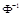(yij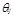-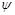(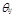))+c(yij,)]

2. The random part conditional on random effects bi, y’ijs are independent random variables with conditional densities belonging to exponential dispersion family and have conditional means and variance

3. The link function which is defined as h E (yiji)=xTijβ+zijbi. Here, h is called the link function and xij and zij are p and q vectors of known covariates. β is a p-dimensional vector of unknown fixed regressor coefficients and bi~N(0, D). Since our response variable is binary, we show this illustration using logistic regression model;

Logit Pr (yij=1/µi)=β0i1xij

This model shows that each individual in our data is exposed to own probability of a normal response (y=1) which is given by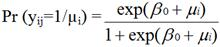The model also indicates that an individual’s odds of a normal response are multiples of exp (β1). The basic principle of the random effects model is that there exists a natural heterogeneity among subjects in a subset of the regression coefficients e.g. in the intercepts. The fundamental assumptions of the random effects model is that b’is are independent of the explanatory variables.

There are certain assumptions that are made in random effects models:-

1. The conditional distribution of yij given bi follow a distribution from the exponential family of distributions with pdf f(yij/bi; β).

2. Given µi, the clustered observation yi1, yi2,…., yni, are independent

3. The bi’s are independent and identically distributed.

2.5. Maximum Likelihood Estimation

In maximum likelihood estimation, bi variables from a random effects distribution. This assumption suggests that by understanding the variability of the overall population, we can learn about an individual’s coefficient. Here, the likelihood function for the unknown parameter δ, which is defined to include both β and elements of G, where bi~i.i.df(µi, G) is: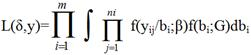This is simply the marginal distribution of Y obtained by integrating the joint distribution of Y and b with respect to b. The maximum likelihood is found by solving the score function which we obtain by setting the first derivative of the likelihood function above with respect to δ to 0.

The complete data score for β has the form;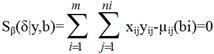Where µij(bi)= E(yij|bi)=ƞ¯1(x’ij+d’ijbi)

These observed data score equations are obtained by taking the expectation of the complete data equations with respect to the conditional distribution of the unobserved random effects given the data. The score function for G is given as;2.6. Logistic Regression for Binary Data

Considering the nature of the response variable in this study, we introduce literature behind logistic regression models as a parametric tool for modeling binary data. Logistic regression models are the most widely used models for categorical response data.

Consider the explanatory variable X of a binary response variable Y and let

π(x) = prob (Y = 1|X = x) = 1- prob (Y =0|X = x)

This yield to the logistic regression model;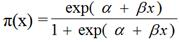In this model the log-odd, which are also called the logits has the linear relationship given by;which is the logit link function to the linear predictor. The sign of the β (log odds) determines the slope of the curve i.e. whether π(x) is falling or rising. For quantitative x with β > 0, the curve of π(x) has the shape of the cumulative distribution function of the logistic distribution, and since the logistic distribution is symmetric, then the π(x) approaches 0 and 1 at the same rate.

Taking exponent of the above equation we get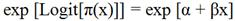This shows that the odds ratios are exponential functions of x. Therefore, the odds increases multiplicatively by eβ for every 1-unit increase in x. i.e. eβ is an odds ratio, the odds at X = x+1 divided by the odds at X = x.

2.7. Inference in Logistic Regression

Wald (1943) showed that the parameter estimators in logistic regression models have (asymptotic) large-sample normal distributions. Thus, inference in logistic regression models can use the Wald, likelihood-ratio methods.

For the model with predictor Logit [π(x)]=𝛼+βx we test the null hypothes is H0:β=0 against H1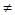0. The wald test uses the log likelihood at β, with the test statistics being z=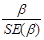. The likelihood ratio test has a χ2 distribution with 1 degree of freedom and uses twice the difference between the maximized log likelihood at β and at β=0. One way of checking for the model fitness is by using the likelihood ratio test to compare the fitted model with a more complex model. Another way of checking for model fit is by checking for any way that the model fails. This procedure checks for the model’s lack of fit other than model fit.

2.8. Mixed Effects Models for Binary Data

In marginal modeling and marginal distributions of clustered responses, the joint dependence structure is treated as a nuisance. There is an alternative approach of using cluster-level terms in the model. These terms are unobserved, taking different values for observations in different clusters. They are treated as varying randomly, hence are called random effects. Random effects models for normal responses are well established and only recently have random effects been used much in models for categorical data. Due to the nature of our outcome variable, we shall narrow this to logistic-normal model. Random effects models for categorical clustered data in an ordinary linear model, fixed effects refer to parameters that describe a factor’s effects and they apply to all categories of interest. Generalized linear models extend ordinary regression by allowing non-normal responses and a link function of the mean, while GLMMs allows random effects as well as fixed effects in the linear predictor.

2.9. The Model

If we let yit denote observation tin cluster i, t=1,.., Ti. We further let xit denote a column vector of values of explanatory variables, for fixed effect model parameters β. Again, let µi denote the vector of random effect values for cluster i. This is common to all observation in a specific cluster. Let zit donate column vector of their explanatory variables. Conditional on µi, a GLMM resembles an ordinary GLM. The linear predictor for the model is defined as;

g(µit)=xTitβ+zTµi

Where the mean µit= E(Yiti) and g(.) is the link function. It’s further assumed that µi~N(0, ∑). We shall introduce here the inter-class and the intra-class correlation in mixed effects model. The intra-class correlation is given by: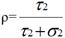Where τ2 is the within group variation, and σ2 is the overall variation, i.e. residual error. The variability of among µi induces a non-negative correlation for the marginal distribution that is averaged over the subjects. Observations within the same cluster i share the same mean µi. Random effects also enter into our model as any other explanatory variables. The purpose of including random effects in a model include among others;

They at times will represent the heterogeneity in the data that is caused by not observing certain predictors. Therefore, random effects model the unobserved predictors by reflecting these terms that would have been in the model.

They provide a way of explaining the over-dispersion in basic models that do not have these effects.

They reflect terms that would otherwise be in the fixed effects part of the model if certain predictors would be included in the model.

They represent random measurement errors in the in dependent variables.

2.10. Binary Responses

The univariate random effect model is of the form;

logit (P[Yit = 1/µi]) = xTit β + µit

Where µi independent ~ N (0, σ2) variates. This model is a special case of a generalized linear mixed model and g(.) is the usual logit link function. Let Ф denote the cumulative density function (cdf) that is the inverse link function. Then, for any st,You shall notice that both Ф (xTisβ + µi) and Ф (xTitβ + µi) are monotonically increasing with µi, therefore are non-negatively correlated. At each t, the predictor variable j pdf of x is interchangeable for clustered data, a factor that is common also with longitudinal data, where observations in close together time wise are likely to be more correlated than observations that are further apart. In estimation, the interpretation is a around the fixed effects, with the random effects used for example, σ the estimate of the standard deviation of the random intercept may be used to predict the population’s degree of heterogeneity.

σ = 0-The model simplifies to a logistics regression model, with all observations independent of each other. Recall the log odds ratio given by;

logit [P (Yit= 1|ui)] – logit[P(Yhs = 1|µh)] = (xit– xhs)Tβ + (µi - µh)

recall that (µi- µh) ~ N(0, 2σ). Thus, 100(1-α)% of the log odds fall with the following range;σ >0 – the log-odds ratio of two observations in same cluster

3. Results

3.1. Introduction

Statistical tools for Microsoft excel, SPSS and R were used for data input and analysis. Some of the explanatory variables were categorized before starting the analysis into two or more categories to make the analysis and interpretations more meaningful. Exploratory data analysis is done using SPSS and R, data is then fed into models for further analysis.

3.2. Variable Descriptions

1. Diseased:-This is a binary variable defined as 1 if an environmental health related disease occurred or 0 if it didn’t occur to an individual

2. Gender:- sex of an individual-coded as 1=Male, 0=Female thus it’s a categorical variable with 2 levels

3. Highest education attained:-it’s a categorical variable with four levels coded 0=None, 1=primary, 2=secondary and 4=tertiary

4. Current working status:-A categorical variable coded 1=working and 0=Not working.

5. Area of Residence:- A categorical variable with 1=Rural and 0=Urban

6. Main source of drinking water:-is a categorical variable coded 1-safe drinking water and 0=unsafe drinking water

7. Human waste disposal:-A binary variable defined as 1 if one use hygienic human waste disposal means or 0 if not

8. Housing condition:-is a binary variable coded 1 if has good housing condition and 0 if has poor housing condition

9. Clust:-clustering variable

3.3. Modeling Individual Morbidity Using Generalized Linear Mixed Model

We fit a GLMM effect model to the individual morbidity data described above. The dependent variable is "diseased", as a measure of whether an individual experienced environmental health related disease.

The generalized linear mixed effects model with logit link is defined as below:

Logit [Pr(yij=1|µi)]=β0i+βixij

The model takes the form;

logit[(Prob(diseasedij)=1|µi)]=β0i1(gender)ij+β2(education)ij+………+β7(housing)ij+b0(clust).

We begin by showing the distribution of different dependent variables. The table below shows all the variables that were fitted in the model.

3.4. Exploratory Data Analysis

In this section we seek to show the distribution of the dependent variable compared to some selected covariates.

Table 1. Summary statistics (Categorical variables)

 Variable Category Descriptive Percentage Gender Male 32,918 49.3 Female 33,807 50.7 Area of residence Rural 47,126 70.9 Urban 19,351 29.1 Working status Working 14,895 66.9 Not working 7,374 33.1 Source of drinking water Protected source 32,870 50.1 Unprotected source 32,732 49.9 Human waste disposal Hygienic waste disposal 31,158 47.5 Unhygienic waste disposal 34,495 52.5 Highest Education None 28,731 61.1 Primary 9,920 21.1 Secondary 4,896 10.4 Tertiary 3,505 7.4 Housing condition Good housing condition 38,788 59.3 Poor housing condition 26,670 40.7

Table 2. Cross-tab of all covariates against the dependent variable "diseased"

 Variable Level Non diseased diseased Total Cramer's V Gender Male 50.3 45.6 49.3 0.037 Female 49.7 54.4 50.7 Area of residence Rural 71.1 70.1 70.9 0.009 Urban 28.9 29.9 29.1 Working status Working 66.4 69.2 66.9 0.023 Not working 33.6 30.8 33.1 Main source water Protected source 49.8 51.2 50.1 0.011 Unprotected source 50.2 48.8 49.9 Human waste disposal Hygienic waste disposal 46.9 49.5 .5 0.02 Unhygienic waste disposal 53.1 50.5 52.5 Highest education None 60.4 64.2 61.1 0.033 Primary 21.4 19.5 21.1 Secondary 10.7 8.9 10.4 Tertiary 7.5 7.4 7.4 Housing condition Good housing condition 59.1 59.8 59.3 0.005 Poor housing condition 40.9 40.2 40.7

3.5. Model Fitting

We fit a GLMEM using the Imer command in R which contains functions for estimation of multilevel or hierarchical regression models. β represents the coefficients of fixed effects while b’s represent the coefficients of the random part.

A generalized linear mixed effect model for all explanatory variables in R produced the model in the table 3 below.

Table 3 shows the fitted GLM with outcome "diseased". This model uses a logit link to estimate the factors that drive morbidity incidences. We use this model to compare the results from the GLMEM reported previously.

Table 3a. Model 1 Null linear mixed model by REML.

 AIC BIC Log Link Deviance REML dev 63774 63792 -31885 63857 63770

Table 3b. Random effects.

 Groups Name Variance Std. Dev Clusters Intercept 0.011986 0.10948 Residual 0.147679 0.38429

Table 3c. Fixed effects.

 Estimate Std. Error z value Pr(>|z|) Intercept 1.53199 0.02271 67.45 <2e-16***

The above model is an empty model i.e. model fitted without including the explanatory variables. The variance component corresponding to the random intercept is 0.011986.

The two variance components can be used to partition the variance across levels. The interclass correlation coefficient is equal to;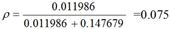meaning that roughly 0.08% of the variance is attributed to the cluster-level. The strength of the intra-cluster correlation determines show observations within a given cluster are likely to be similar to each other. Thus, a higher intra-cluster correlation gives a more pronounced "clustering effect."

To explain some of the cluster-level variance, we incorporate the explanatory variables in the empty model. The table below shows the GLMM for the random intercept and fixed predictors in individual level using REML.

Table 4. Model 2: GLMM for the random intercept and fixed predictors using REML.

 AIC BIC Log link deviance REML dev 3946 4023 -1961 3855 3922 Random effects Groups Name Variance Std. Dev Clusters Intercept 0.0077387 0.08797 Residual 0.1393540 0.37330 Fixed effects Estimates Std. Error z value Pr(>|z|) (intercept) 1.35917 0.13153 10.334 <2e-16*** Male 0.26122 0.08322 3.139 0.00170 Urban -0.11217 0.13598 -0.825 0.40943 Working -0.06090 0.09769 -0.623 0.53304 Unprotected water source 0.01772 0.10003 0.177 0.85938 Unhygienic waste disposal 0.10290 0.09925 1.037 0.09984 Primary -0.01127 0.10086 -0.112 0.91103 Secondary 0.15345 0.13454 1.141 0.25407 Tertiary 0.04185 0.12827 0.326 0.74420 Poor housing condition 0.23273 0.10640 2.187 0.02872

The variance component corresponding to the random intercept has decreases to 0.0077387, indicating that the inclusion of the explanatory variables has accounted for the some of the unexplained variance. Comparing both the AIC and BIC statistics in both models above, it is clear that the model 2 is preferable to the model 1since it gives smaller values of AIC and BIC.

From the GLMM model above; gender, human waste disposal and housing condition are significant in predicting the probability of an individual getting an environmental health related disease. However, area of residence, education and working condition and main source of water are insignificant.

The GLMM model is of the form

logit [(prob(diseasedij) = 1/µi] = β0 + µi + β1(gender)ij + β2(human waste disposal)ij + β3(housing condition)ij

The GLMM outputs above indicates that with group of the female as the reference group; then the log of odds of getting an environmental health related disease increases by 0.0017.

Holding other variables constant; an individual living in poor housing condition is about 3% more likely to have the disease compared to an individual living in a good housing condition. Also the odds of getting an environmental health related disease is exp (0.09984) = 1.10499 times for unhygienic waste disposal compared to hygienic means of human waste disposal.

Table 5. A generalized linear model for "diseased".

 AIC 4002.792 Estimate Std. Error z value p-value (intercept) 1.232434 0.11169 11.034 2.00E-16 Male 0.24782 0.080393 3.083 0.002052 Urban -0.108088 0.104391 -1.035 0.300476 Working -0.097075 0.09077 -1.069 0.28486 Unprotected source 0.041063 0.083227 0.493 0.001744 Unhygienic waste disposal 0.145195 0.087092 1.667 0.095486 Poor housing condition 0.323525 0.089386 3.619 0.000295 Primary 0.002356 0.096739 0.024 0.980572 Secondary 0.182476 0.12824 1.423 0.154758 Tertiary 0.056151 0.121077 0.464 0.64282

The Akaike Information Criteria (AIC) for GLM model was 4002.792 which is a measure of goodness of fit that takes the number of fitted parameters into account. This value is larger as compared to AIC in the GLMM model. Thus GLMM model is preferable to GLM in modeling clustered data.

From the GLM model above; gender, human waste disposal, housing condition and main source of drinking water are significant in predicting the probability of an individual getting an environmental health related disease. However, area of residence, education and working condition are insignificant.

Hence the GLM model would be

ln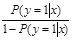01(gender)+β2(housing condition)+β3(main source of water)+β4(human waste disposal)

The GLM outputs above indicates that with group of the female as the reference group; then the log of odds of getting an environmental health related disease increases by 0.24782. For the main source of water variable, the odds of getting an environmental health related disease is exp (0.041063) = 1.0419 times for unprotected main source of water compared to protected source of water. Holding other variables constant; an individual living in poor housing condition is about 38% more likely to have the disease compared to an individual living in a good housing condition. Also the odds of getting an environmental health related disease is exp (0.145195) = 1.1563 times for unhygienic waste disposal compared to hygienic means of human waste disposal.

4. Conclusions, Recommendations and Suggestions for Further Studies

4.1. Conclusions

This study was set to determine factors that are associated with the probability of an individual in a population experiencing an environmental health related disease in Kenya. It was also set to develop a statistical model that describes the influence of these factors while accounting for inter-class correlation in the data. The study found that individual morbidity is associated with some social, economic and demographic factors in the country. The study applied both GLM and GLMM models to model household data that was collected in 2005/6 to investigate factors associated with environmental health related diseases. Further, the study applied Akaike Information Criteria (AIC) to determine the preferable model in modeling clustered data.

From the analysis, it was found that, these verity of environmental health related disease is likely to increase with gender where by a female individual is likely to get a disease than a male individual. This outcome supports the idea that gender-specific differences in morbidity and mortality may be explained by genetic factors and by their differential response to the environment.

People living in poor housing conditions were found to be more likely to get a disease than those from good housing condition. Main source of drinking water was also significant in explaining individual morbidity in Kenya with an individual using unprotected main source of water found more likely to get a disease than an individual using protected main source of water.

Means of human waste disposal was another factor found affecting the disease outcome where by an individual using unhygienic waste disposal was more likely to have an environmental health related disease than the one using hygienic means.

However, the study found that area of residence; working condition and education level do not affect the diseased outcome.

On the statistical model that account for inter-class correlation in the data, it was found that the value of AIC in GLM model was larger compared to AIC value in GLMM model. According to Akaike’s theory, the most accurate model has the smallest AIC hence; for this study, it could conclude that GLMM model is more preferable to GLM in modeling clustered household data.

4.2. Recommendations

Efforts to address the plight of the environmental health related disease should be more focused to individuals living in poor conditions and should not only be focus in offering facilities but also economic empowerment.

4.3. Suggestions for Further Studies

This study can be extended to incorporate income level of individuals. Individuals with low level of income are believed to be more likely to experience environmental health related diseases than individuals with higher levels of income.

Further studies should also be carried out to focus on mapping the areas which are mostly affected in the country and developing an effective model to address the issue.

References

1. Cande V Ananth, Robert W Platt (2004) Re-examining the effects of gestational age, fetal grow and maternal smoking on neonatal mortality
2. Alan Agresti (2002), Categorical Data Analysis. 12, pg 491-537
3. Katrien Antonio, Jan Beirlant (2006) Acturial Statistics With Generalized Linear Mixed Models
4. Daowen Zhang (2004) Generalized Linear Mixed Models with Varying Coefficients for Longitudinal data
5. Artazcoz L, Benach J, Borrel C, Cortes I 2004 Unemployment and mental health: understanding the interactions among gender, family roles and social class. American Journal of Public Health 94: 82–88
6. Wolfinger, R., and M. O’ Connell. 1993. "Generalized Linear Mixed Models: A Pseudo-Likelihood Approach. Journal of Statistical Computation and Simulation 48: 233-43.
7. Gene A. Pennello, Susan S. Devesa, and Mitchell H. Gail (1999) using Mixed effects model to Estimate Geographic Variation in Cancer Rates
8. Lei Nei (2005) Convergence rate of MLE in Generalized Linear and Non Linear Mixed-effect Models: Theory and Applications
9. D. I Ohlssen, L. D Sharples, and Spiegel halter (2000) Flexible random effects models using Bayesian semi-parametric models: application to institutional comparisons
10. Petra Bukovand Thomas Lumley (2007). Longitudinal Data Analysis for Generalized Linear Models with Follow-up Dependent on Outcome-Related Variables. The Cana-dian Journal of Statistics, Vol. 35, No. 4, pp. 485-500
11. James A. Hanleyon (2002) Statistical Analysis of Correlated Data Using Generalized Estimating Equations: An Orientation.
12. Maxwell, S. E. & Delaney, H. D. (2004). Designing Experiments and Analyzing Data: A Model Comparison Perspective, Second Edition. Mahwah, NJ: Lawrence Erlbaum Associates, Inc.
13. Hayes, W. L. (1973). Statistics for the Social Sciences. New York: Holt, Rinehart, & Winston
14. American Journal of Theoretical and Applied Statistics 2015; 4 (3): 170-177177
15. Mohammed O. M. Mohammed. Statistical methods for analyzing complex survey data: An application to morbidity in ethiopia. 2013.
16. G Rasch. On general laws and the meaning of measurements in psychology in Neyman J, ed. Proceedings of the 4th Berkeley symposium on mathematical statistics and probability, vol 4. Berkeley, 1961.

 Contents 1. 1.1. 1.2. 2. 2.1. 2.2. 2.3. 2.4. 2.5. 2.6. 2.7. 2.8. 2.9. 2.10. 3. 3.1. 3.2. 3.3. 3.4. 3.5. 4. 4.1. 4.2. 4.3.
Article ToolsAbstractPDF(522K)